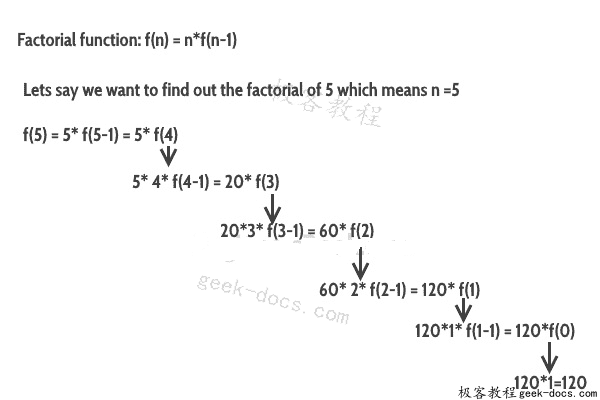# C++ 递归## C++ 递归示例：阶乘

#include <iostream>
using namespace std;
//Factorial function
int f(int n){
/* This is called the base condition, it is
* very important to specify the base condition
* in recursion, otherwise your program will throw
* stack overflow error.
*/
if (n <= 1)
return 1;
else
return n*f(n-1);
}
int main(){
int num;
cout<<"Enter a number: ";
cin>>num;
cout<<"Factorial of entered number: "<<f(num);
return 0;
}


Enter a number: 5
Factorial of entered number: 120


### 基本情况

if (n <= 1)
return 1;


## 直接递归与间接递归

### C++ 中的间接递归示例

#include <iostream>
using namespace std;
int fa(int);
int fb(int);
int fa(int n){
if(n<=1)
return 1;
else
return n*fb(n-1);
}
int fb(int n){
if(n<=1)
return 1;
else
return n*fa(n-1);
}
int main(){
int num=5;
cout<<fa(num);
return 0;
}


120


• 回顶